# Equilateral polygon

In geometry, three or more than three straight lines (or segment of a line) make a polygon and an equilateral polygon is a polygon which has all sides of the same length. Except in the triangle case, it need not be equiangular (need not have all angles equal), but if it does then it is a regular polygon. If the number of sides is at least five, an equilateral polygon need not be a convex polygon: it could be concave or even self-intersecting.

## Examples

All regular polygons and isotoxal polygons are equilateral.

An equilateral triangle is a regular triangle with 60° internal angles.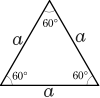An equilateral quadrilateral is called a rhombus, an isotoxal polygon described by an angle α. It includes the square as a special case.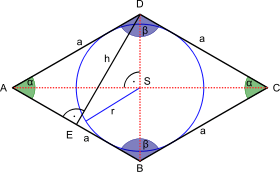A convex equilateral pentagon can be described by two angles α and β, which together determine the other angles. Concave equilateral pentagons exist, as do concave equilateral polygons with any larger number of sides.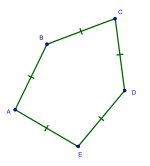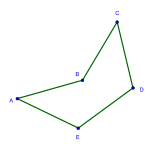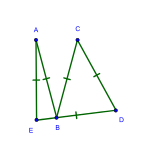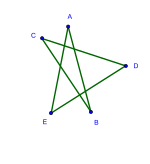An equilateral polygon which is cyclic (its vertices are on a circle) is a regular polygon (a polygon that is both equilateral and equiangular).

A tangential polygon (one that has an incircle tangent to all its sides) is equilateral if and only if the alternate angles are equal (that is, angles 1, 3, 5, ... are equal and angles 2, 4, ... are equal). Thus if the number of sides n is odd, a tangential polygon is equilateral if and only if it is regular.

Viviani's theorem generalizes to equilateral polygons: The sum of the perpendicular distances from an interior point to the sides of an equilateral polygon is independent of the location of the interior point.

The principal diagonals of a hexagon each divide the hexagon into quadrilaterals. In any convex equilateral hexagon with common side a, there exists:p.184,#286.3 a principal diagonal d1 such thatand a principal diagonal d2 such that## Triambi

Triambi are equilateral hexagons with trigonal symmetry:

1. De Villiers, Michael (March 2011), "Equi-angled cyclic and equilateral circumscribed polygons" (PDF), Mathematical Gazette, 95: 102–107.
2. De Villiers, Michael, "An illustration of the explanatory and discovery functions of proof", Leonardo, 33 (3): 1–8, explaining (proving) Viviani’s theorem for an equilateral triangle by determining the area of the three triangles it is divided up into, and noticing the ‘common factor’ of the equal sides of these triangles as bases, may allow one to immediately see that the result generalises to any equilateral polygon.
3. Inequalities proposed in “Crux Mathematicorum, .Wikimedia Commons has media related to Equilateral polygons.